```SIMULATION OF THE DYNAMIC RESPONSE
OF ALUMINUM REDUCTION CELLS
```
```Imad Tabsh, COMPUSIM Inc.
1003D 55 Avenue N.E., Calgary, Alberta, Canada  T2E 6W1

Marc Dupuis, GéniSim
3111 Alger, Jonquière, Québec, Canada  G7S 2M9

```

 Abstract A comprehensive program (ARC/DYNAMIC) was developed to simulate the dynamic behavior of aluminum reduction cells during operation. The program uses the mass and energy balance equations to determine the transient evolution of more than 60 process variables. In addition, it simulates various operational and control policies in use during cell operation. This paper describes the use of ARC/DYNAMIC to study the sensitivity of cell dynamic response to the BEMF. A description of the steady state solver and the amperage fluctuation model implemented in the program is presented. Introduction The dynamic behavior of a reduction cell during operation is a complex phenomenon that is affected by a number of parameters in the pot. A good understanding of this behavior is invaluable in determining a control strategy that optimizes the cell operation and maximizes its efficiency. Mathematical models are valuable tools that help achieve a better understanding of the process and can be used to study "what if" scenarios without disturbing the pot line operation. A computer program, ARC/DYNAMIC, was developed to model the dynamic behavior of reduction cells. A complete overview of the program is described in reference . Briefly, the program solves 13 differential equations that characterize the mass and heat balance in the cell. The behavior within the pot is characterized using 16 dynamic variables and more than 40 variables derived from them. ARC/DYNAMIC calculates the dynamic evolution of the simulation variables over time by modeling the physical process as well as the control algorithms used to operate the cell. This paper will focus on using ARC/DYNAMIC to study the sensitivity of the pot operation to the back electro-motive force (BEMF) used in calculating the pseudo-resistance of the pot. A brief overview of the steady state solver and the amperage fluctuation submodel is presented here since they are extensively used in the study. Steady State Solver A recent addition to ARC/DYNAMIC was a more sophisticated steady state solver as well as the ability to run Monte Carlo simulations to study the sensitivity of the pot response to input variables . The steady state solution, by definition, assumes that the internal heat is equal to the heat lost from the cell, i.e. no melting or growing of freeze and no change in the operating temperature occurs. This condition is often used in the initial design of a cell. The program defines any one of 18 input variables as the target variable to be calculated in a steady state condition. The remaining 17 are specified by the user along with all the other data necessary to characterize the cell model. ARC/DYNAMIC uses a Newton-Raphson algorithm to search for the root of the steady state equation. Any or all of the 17 variables can have a probability distribution assigned to it and a Monte Carlo solution is performed to calculate the distribution of the target variable. A linear distribution of a particular variable (in this study the cell amperage) can be used to create a "response space" for evaluating the pot characteristics. The interaction between any two variables in the response space can be evaluated using a regression analysis. Amperage Fluctuation Submodel The other important feature of ARC/DYNAMIC used in this study is the amperage fluctuation submodel. The purpose of this submodel is to synthesize a cell amperage time history that accounts for the impact on the amperage of anode effects in various pots in the line. The user specifies a probability density function that defines the probability of x pots having an anode effect simultaneously. PBF pots have a distribution that does not vary with time. Side break pots have a distribution which is a function of the feeding cycle. The closer you are to the feeding time the higher is the probability of having more pots with anode effects. This behavior is represented in ARC/DYNAMIC by specifying multiple probability functions each applicable to a fraction of the feeding cycle. Once the number of anode effects is known, the amperage can be calculated knowing the maximum capacity of the rectifier, the target line amperage, and the voltage of each pot. The later is calculated by specifying a constant voltage for pots not in anode effect and a voltage increment for pots in anode effect. The voltage increment is assumed to be a normal distribution with the mean and standard deviation specified by the user. The net result is an amperage distribution over time similar to what is shown in figure 4. This is characteristic of what is observed in real pot rooms. Evaluation of the Back EMF When it is time to regulate the cell ACD to keep the voltage and thereby the internal energy constant, it is common practice to control the so-called pseudo-resistance instead of controlling directly the voltage. This is required to filter out the effect of fluctuations in the line amperage on the

cell voltage. Since the cell voltage was found to vary linearly with amperage, the cell pseudo-resistance (R) is computed as follows:(1)

where U is the cell voltage, I is the line amperage and BEMF is referred to as the back electro-motive force. BEMF is really the zero current intercept of the tangent to the cell voltage vs. cell amperage curve at the point of nominal cell operation. By definition, R should not be affected by the fluctuation in the line amperage.

Factors Affecting the BMF

An evaluation of the factors that affect the BEMF requires a close look at the cell voltage since the BEMF is calculated based on the slope of the voltage versus cell amperage curve. The cell voltage (Ucell) is defined as:

Ucell=Ubath+Uelec+Uext+ Uand+Ucath (2)

where

 Ubath Voltage drop in the bath; Uelec Electrolysis voltage; Uext Average voltage drop in the external busbars; Uand Anode mid life average voltage drop; Ucath Cathode average voltage drop at a given life.

The bath voltage is a function of the bath resistivity and the voltage drop in the bubble layer below the anodes. Models of the bath resistivity have been published in the literature [3,4]. It is a function of the bath ratio, concentration of alumina and other additives in the bath, and the operating temperature. Haupin  expressed the bubble layer voltage as a function of the current density, bath resistance, alumina concentration and the thickness of the bubble layer. Welch  proposed a simpler model which expressed the bubble voltage as a function of the bath resistivity, anode current density, the thickness of the bubble layer and the gas fraction in the layer.

The electrolysis voltage is characterized by four components namely the reversible (equilibrium) voltage, concentration overvoltage at the cathode, reaction overvoltage at the anode and concentration overvoltage at the anode. The components are represented by complex semi-empirical expressions and the reader is referred to the literature for more details [5,6]. The expressions are a function of the current density, bath ratio, alumina concentration and the operating temperature [7,8].

The cathode, anode and external voltage are dictated by the cell design and the busbar configuration. Depending on the desired accuracy, they can be calculated using a simplified network analysis or detailed finite element thermo-electric models .

It is clear from the above that several factors affect the cell voltage and hence the BEMF. The anode and cathode current density play a major role. They are a function of the cell amperage as well as the anode geometry and the freeze profile the later being highly dependent on the cell operation. Two other important factors are the operating temperature and the alumina concentration in the bath.

The BEMF is often assumed to be constant with different values quoted in different sources (1.62-1.68 V in reference  1.6-1.8 V in reference ). A value of 1.65 is often chosen. It is common practice within each smelter to calculate a value of BEMF from the recorded line amperage and cell voltage.

Two examples, a PBF and a side break cell design, will be used to study the values of BEMF and evaluate the effect of its accuracy on the pot behavior.

PBF Cell Operating at 300 kA

As a first case study let us use the PBF prototype cell operating at 300 kA presented in an article in the February 1994 issue of JOM . First, we can use the Monte Carlo mode of the steady state solver to study the impact of the amperage decrease on the cell voltage at constant ACD and cell temperature. To do this, the metal level was selected as the target variable. The metal level is calculated that would allow the cell heat loss to remain equal to the internal heat as the amperage is decreased.

As we can see in Figure 1, for this particular cell design, the zero current intercept or BEMF is equal to 1.632V.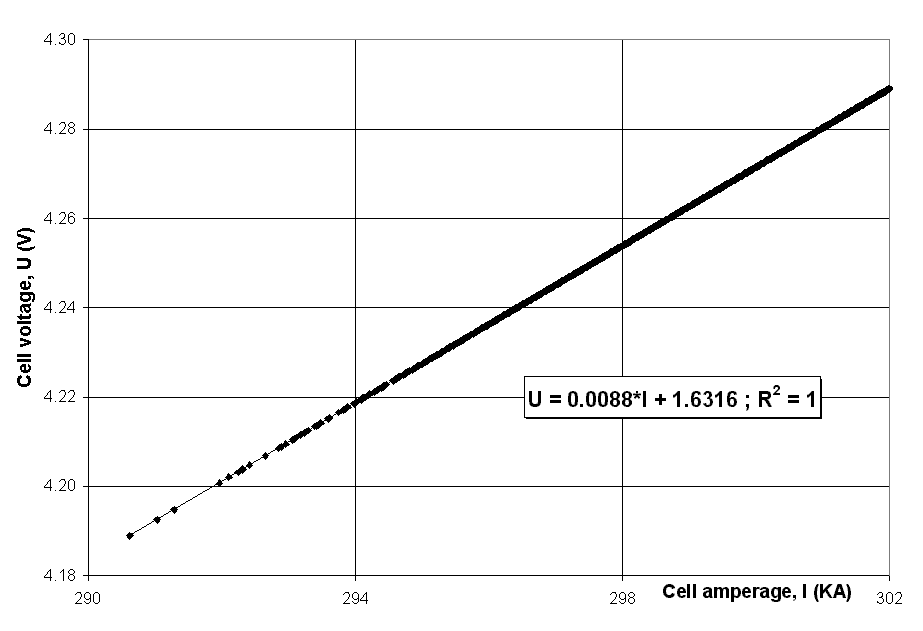Figure 1 - Correlation between the cell amperage and the cell voltage (PBF cell with the metal height as the target variable).

To help illustrate the sensitivity of the value of the BEMF, let us change the setup of the steady state Monte Carlo solution. This time we will keep the ACD constant, but we will select the temperature as the target variable to converge the heat balance as the amperage decreases.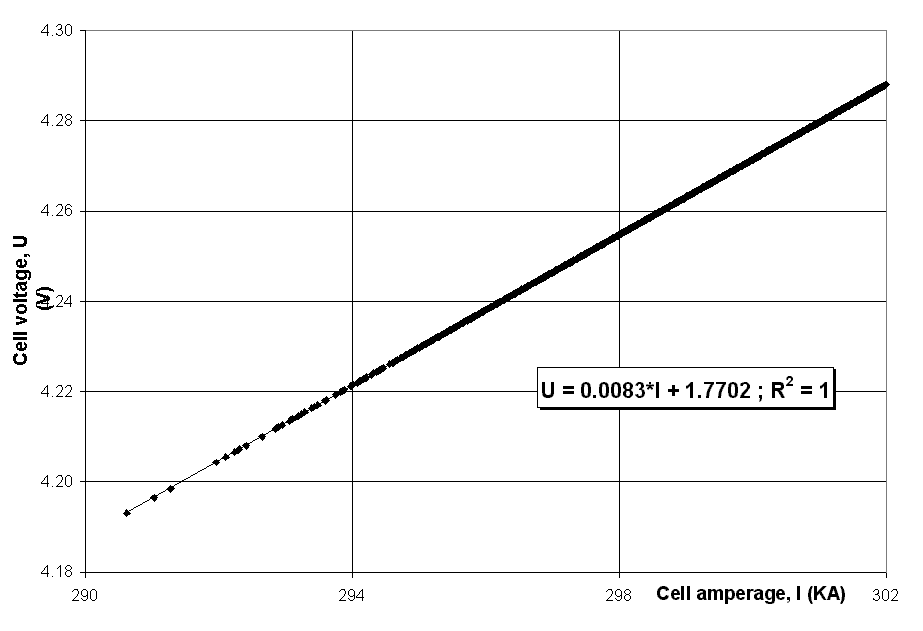Figure 2 - Correlation between the cell amperage and the cell voltage (PBF cell with the cell temperature as the target variable).

With this setup, the value of the zero current intercept is increased to 1.770V (see figure 2). A decrease in temperature, as shown in figure 3, will cause an increase in the bath resistivity and hence an increase in the cell voltage. This increase is offset by the reduction in voltage due to the

 reduction in cell amperage. The net effect is an upward shift in the curve resulting in the higher intercept. Hence, one has to be careful in the selection of the target variable not to impact the cell resistance and generate the wrong result.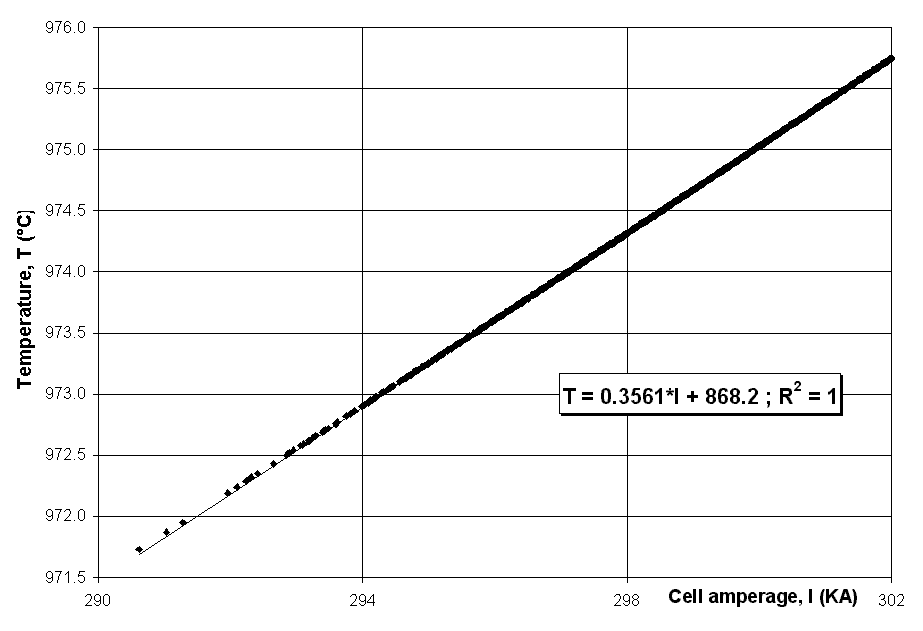Figure 3 - Correlation between the cell amperage and the cell temperature (PBF cell with the cell temperature as the target variable) More importantly, one has to be careful what experimental data should be used to calculate the BEMF. The wrong information may lead to quite a different conclusion. Efficiency of the BEMF to Filter Amperage Noise It is unrealistic to assume that the temperature dynamic evolution can follow line amperage fluctuation. Hence, the best possible value of the BEMF to use in calculating the cell pseudo-resistance is 1.632V. We will now run the model in dynamic mode with and without amperage fluctuation activated. We will also use the "standard" value of 1.65V for the BEMF (assuming that we don´t know better). We want to investigate how well we are able to filter out the impact of the amperage fluctuation on the dynamic evolution of the cell pseudo-resistance. Figure 4 shows the amperage variation with time as calculated using the amperage fluctuation submodel. The nominal current of 300 kA is also shown on the figure. This curve is typical of the fluctuations recorded in operating pot lines.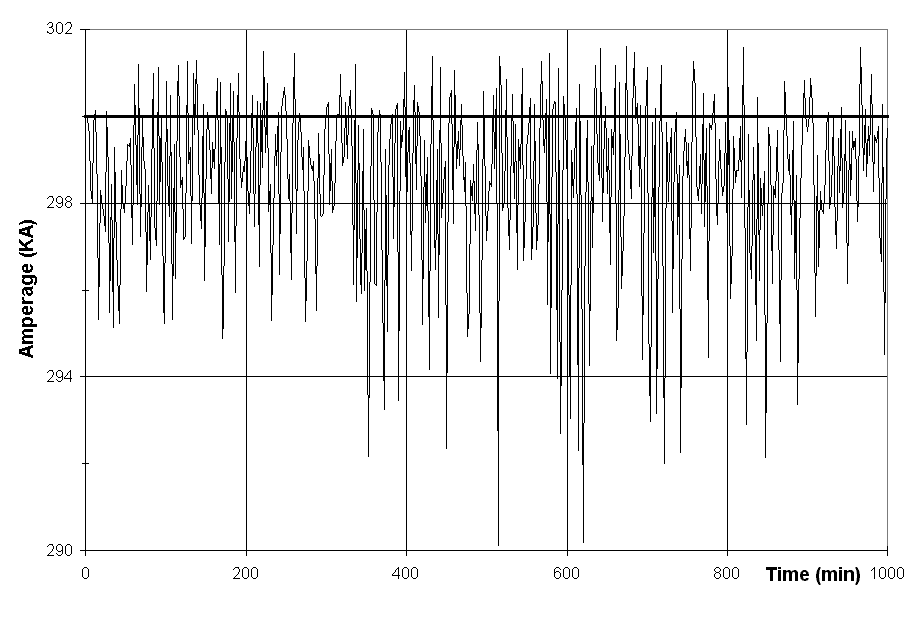Figure 4 - Dynamic evolution of the cell amperage (PBF cell with BEMF=1.65V). As we can see in figure 5, the dynamic evolution of the cell voltage is affected by the amperage fluctuation. On the other hand, results in the top of figure 6 indicate clearly that even with a non optimum value of 1.65 for the BEMF, the effect of the amperage fluctuation has been almost completely removed from the cell pseudo-resistance dynamic evolution. Figure 6 also shows the pseudo-resistance calculated using a BEMF value of 1.770V. Notice that the amperage fluctuation effect is filtered out reasonably well even at such a large deviation from the optimum value. Note, however, that the filtering is not as efficient as the previous case. Furthermore, the average resistance is lower in view of the higher BEMF value (see equation (1)).Figure 5 - Dynamic evolution of the cell voltage (PBF cell with BEMF-1.65V).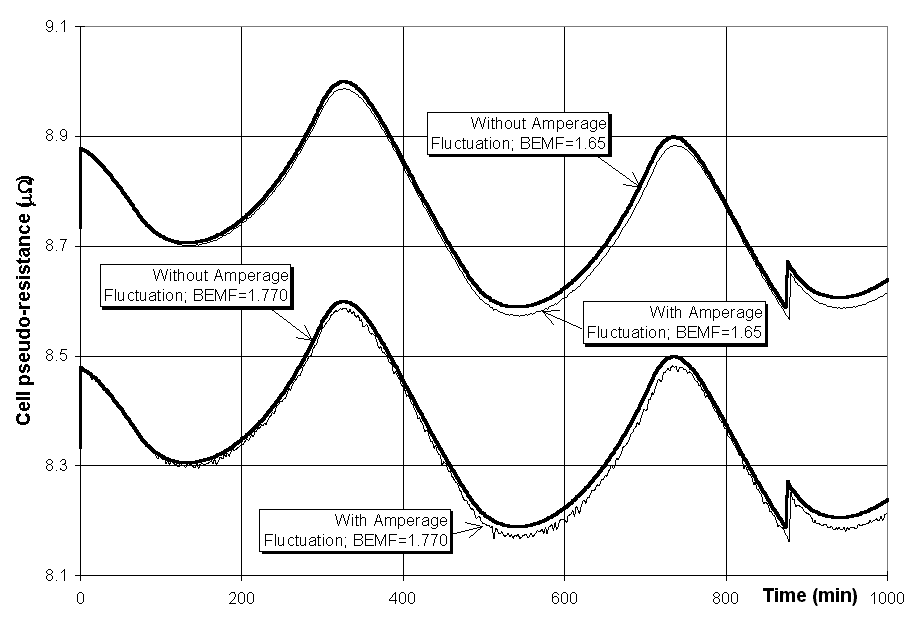Figure 6 - Dynamic evolution of the cell pseudo-resistance (PBF cell w/ and w/o amperage fluctuation for a BEMF of 1.65V (top curves) and 1.77V (bottom curves)). The above data suggests that there is a wide margin of error in selecting the BEMF in PBF cells provided that you are operating close to the nominal cell amperage. The important point to keep in mind is that you do not need a perfect noise filter. Instead, what is required is a BEMF value that reduces the pseudo-resistance fluctuation to a level well below the noise from the ACD fluctuation due to the metal roll and gas bubbles under the anode. It is important not to confuse the two effects and try and kill a non-existing instability in the pot.

 Side Break Söderberg Cell As second case study we will use the side break Söderberg cell operating at 120 kA presented as the validation test case of the dynamic model . Side broken cell technology typically suffers from greater amplitudes of amperage fluctuation due to the higher incidence of anode effects, so let us verify if the above conclusions are valid in this case. The variation of cell voltage with amperage is shown in figure 7. The first major difference you see is that the BEMF characteristic of this cell design is 1.587V which is significantly lower than the 1.65V quoted value. This confirms the view that the BEMF does vary with cell technology and the variation can be significant.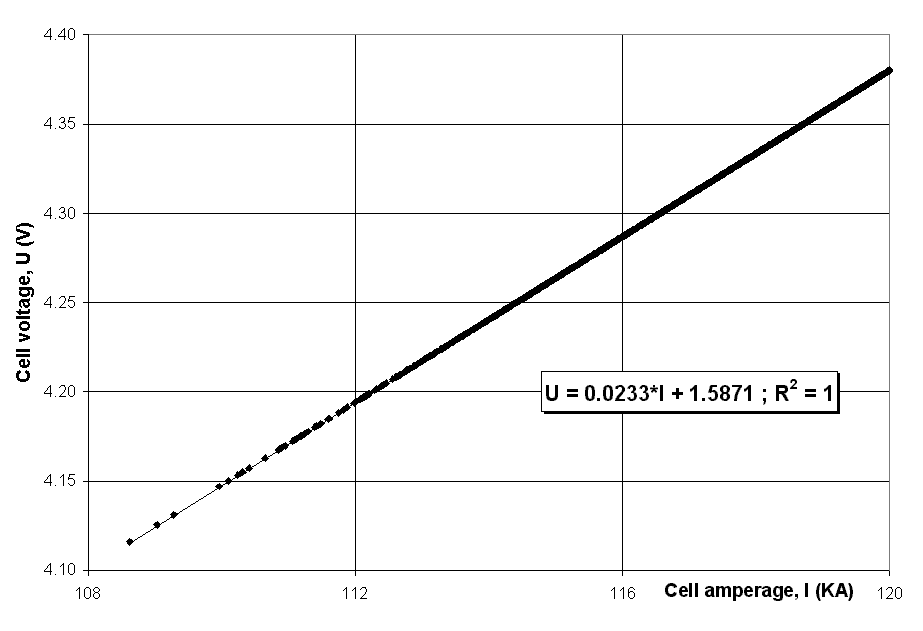Figure 7 - Correlation between the cell amperage and the cell voltage (Side break cell with the metal height as the target variable). We now look at the efficiency of the BEMF to filter the amperage noise. Again, we will first compare the dynamic evolution of the cell pseudo-resistance with and without amperage fluctuations when the BEMF is set to 1.65V.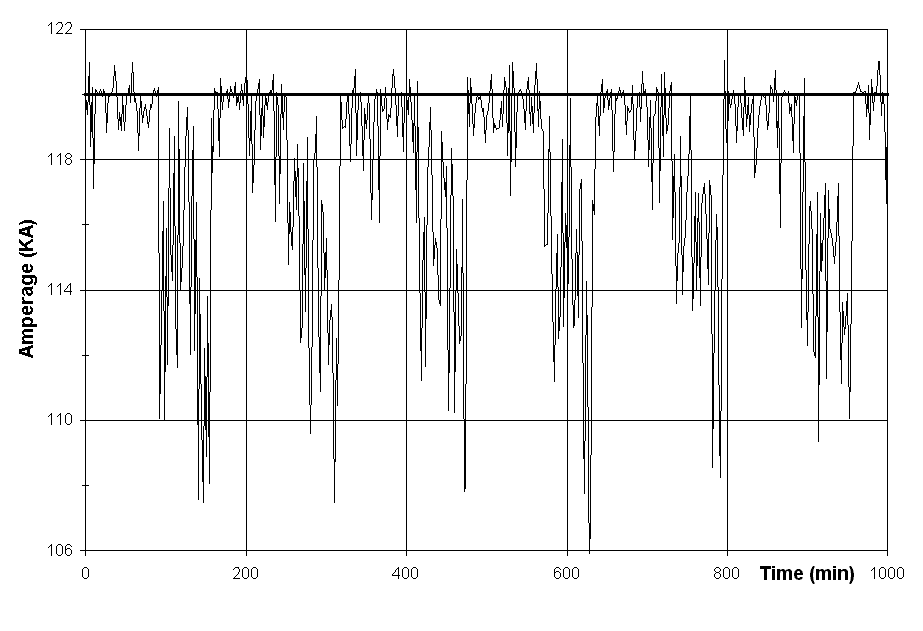Figure 8 shows the amperage fluctuation over time synthesized by ARC/DYNAMIC based on the probability distributions defined for the model. Notice that for the side break cell technology, the amperage fluctuation is characterized by a cyclic pattern that corresponds to the potroom side break feeding cycle. Furthermore, we notice a significant drop in the line current at the end of the feeding cycle when the probability of having many cells in the line in anode effect is high and the rectifiers have reached their maximum power output. The evolution of the cell voltage and the pseudo-resistance with and without amperage fluctuation are shown in figures 9 and 10 respectively. The smooth solid line shows the case of no amperage fluctuation.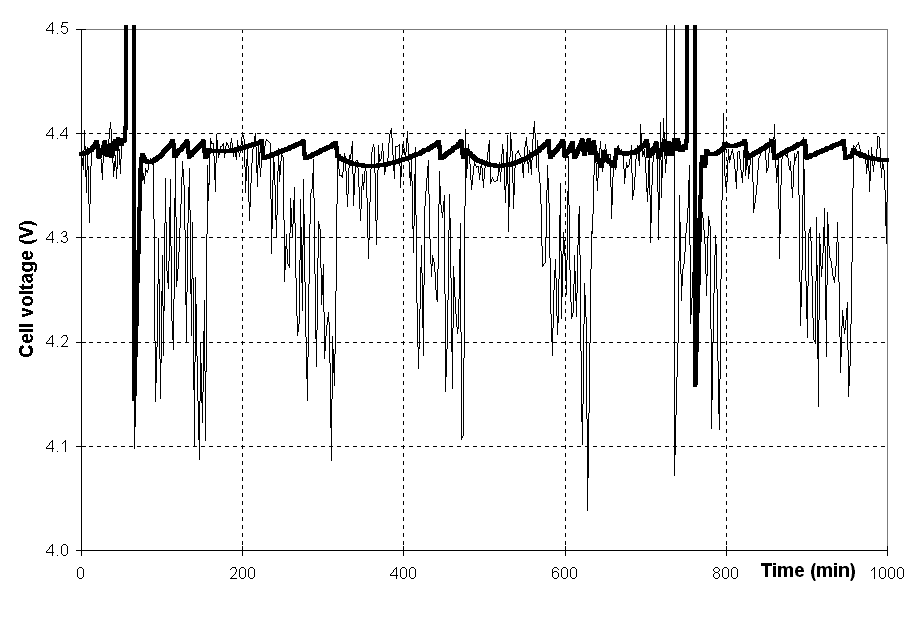Figure 9 - Dynamic evolution of the cell voltage (Side break cell with BEMF=1.65V). This time, as we can see in figure 10, the amperage fluctuations are significantly affecting the dynamic evolution of the cell pseudo-resistance.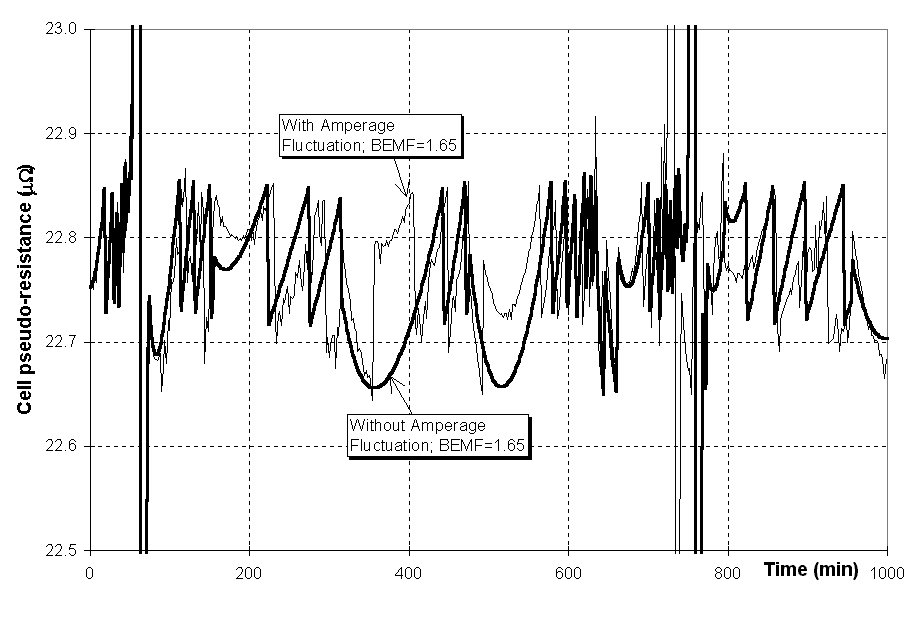Figure 10 - Dynamic evolution of the cell pseudo-resistance (Side break cell w/ and w/o amperage fluctuation for a BEMF of 1.65V).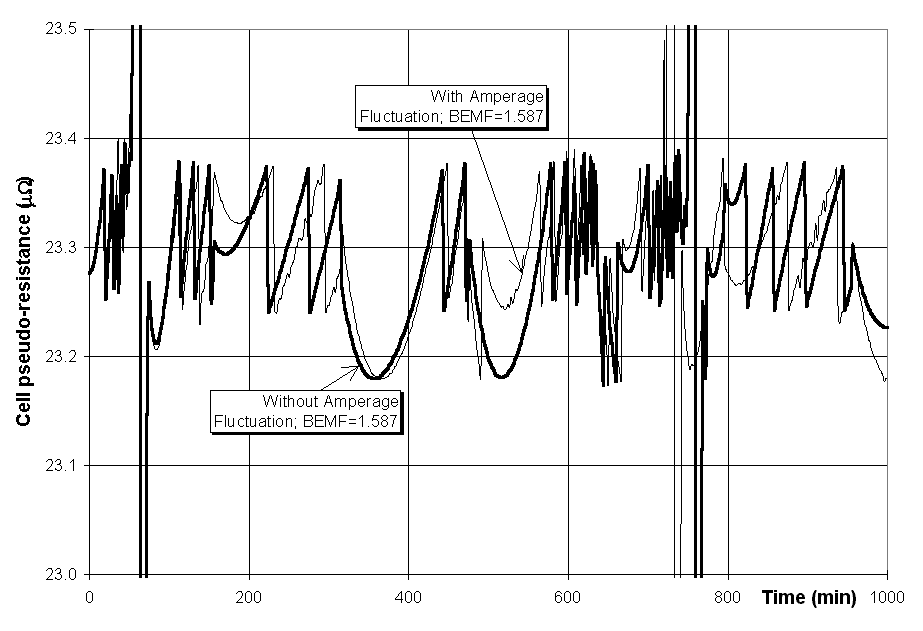Figure 11 - Dynamic evolution of the cell pseudo-resistance (Side break cell w/ and w/o amperage fluctuation for a BEMF of 1.587V).

 To see if the situation can be improved, we will rerun the model in dynamic mode but this time with BEMF set to calculated value of 1.587V. As we can see in figure 11, using the optimum BEMF value has improved the situation. Obviously, this has also changed the steady state value of the cell pseudo-resistance so the cell target resistance had to be readjusted accordingly. It is clear from the above that side break technology is more sensitive to the value of the BEMF in filtering the amperage fluctuations. Conclusions The ARC/DYNAMIC models have shown that the pseudo-resistance calculated using the BEMF is an efficient mechanism for filtering out the amperage fluctuations due to anode effects. Furthermore, PBF cell technology is more forgiving than side break cells when the wrong value of BEMF is used. Finally, the procedure for calculating the BEMF value is important especially when this is done using experimental data. References I. Tabsh and M. Dupuis, "Process Simulation of Aluminum Reduction cells", Light Metals, (1996), 451-457. M. Dupuis and I. Tabsh, "Using a Steady State Model of an Aluminum Reduction Cell to Investigate the Impact of Design changes", Light Metals ’96, Proceedings of the International Symposium, Edited by M. Avedesian, R. Guilbault and D. Ksinsik, 419-429. X. Wang, R.D. Peterson and A.T. Tabereaux, "A Multiple Regression Equation for the Electrical Conductivity of Cryolite Melts", Light Metals, (1993), 247-255. G. Choudhary, "Electrical Conductivity for the Electrolytes of Aluminum Extraction Cell", J. Electrochem. Soc., 120(3) (1973), 381-383. W. Haupin, "Cell voltage Components", CMP/PCPE Course on Aluminum Electrolysis, (1994). Introduction to Aluminum Electrolysis, K. Grjotheim and H. Kavande (Editors), 2nd Edition, Aluminium-Verlag, Düsseldorf, 1993. E.W. Dewing, "The Chemistry of the Aluminum Reduction Cell", Can. Met. Quart., 13(4)(1974), 607-617. A. Solheim, S. Roselth, E. Skybakmoen and L. Støen, "Liquidus Temperature and Alumina Solubility in the System Na3AlF6-AlF3-LiF-CaF2-MgF2", Light Metals, (1995), 451-460. I. Tabsh and M. Dupuis, "Modeling of Aluminum Reduction Cells Using Finite Element Analysis Techniques", Light Metals, (1995), 295-299. Aluminum Smelter Technology, K. Grjotheim and B.J. Welch, 2nd Edition, Aluminium-Verlag, Düsseldorf, 1988. V.A. Kryukovski, G.A. Sirasutdinov, J. Klein and G. Peychal-Heiling, "International Cooperation and High-Performance Reduction in Siberia", JOM, 42(2) (1994), 23-25.